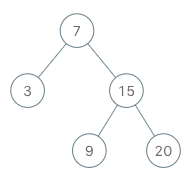# 173. Binary search tree iterator

Binary Search Tree Iterator - LeetCode

Implement the BSTIterator class that represents an iterator over the in-order traversal of a binary search tree (BST):

``````BSTIterator(TreeNode root) Initializes an object of the BSTIterator class. The root of the BST is given as part of the constructor. The pointer should be initialized to a non-existent number smaller than any element in the BST.
boolean hasNext() Returns true if there exists a number in the traversal to the right of the pointer, otherwise returns false.
int next() Moves the pointer to the right, then returns the number at the pointer.Notice that by initializing the pointer to a non-existent smallest number, the first call to next() will return the smallest element in the BST.
``````

You may assume that next() calls will always be valid. That is, there will be at least a next number in the in-order traversal when next() is called.

Example 1:Input [“BSTIterator”, “next”, “next”, “hasNext”, “next”, “hasNext”, “next”, “hasNext”, “next”, “hasNext”] [[[7, 3, 15, null, null, 9, 20]], [], [], [], [], [], [], [], [], []] Output [null, 3, 7, true, 9, true, 15, true, 20, false] Explanation BSTIterator bSTIterator = new BSTIterator([7, 3, 15, null, null, 9, 20]); bSTIterator.next(); // return 3 bSTIterator.next(); // return 7 bSTIterator.hasNext(); // return True bSTIterator.next(); // return 9 bSTIterator.hasNext(); // return True bSTIterator.next(); // return 15 bSTIterator.hasNext(); // return True bSTIterator.next(); // return 20 bSTIterator.hasNext(); // return False

Constraints:

``````The number of nodes in the tree is in the range [1, 105].
0 <= Node.val <= 106
At most 105 calls will be made to hasNext, and next.
``````

``````Could you implement next() and hasNext() to run in average O(1) time and use O(h) memory, where h is the height of the tree?
``````

• code
``````class BSTIterator {
ArrayList<Integer> list;
int index;
private void inorder(TreeNode node){
if (node == null) return;
inorder(node.left);
inorder(node.right);
}

public BSTIterator(TreeNode root) {
list = new ArrayList<>();
index = -1;
inorder(root);
}

public int next() {
return list.get(++index);
}

public boolean hasNext() {
return index < list.size() - 1;
}
}
``````
• code
``````class BSTIterator:

def __init__(self, root: Optional[TreeNode]):
self.stack = []
self.inorderLeft(root)

def inorderLeft(self, node):
while node:
self.stack.append(node)
node = node.left

def next(self) -> int:
cur = self.stack.pop()
self.inorderLeft(cur.right)
return cur.val

def hasNext(self) -> bool:
return self.stack
``````